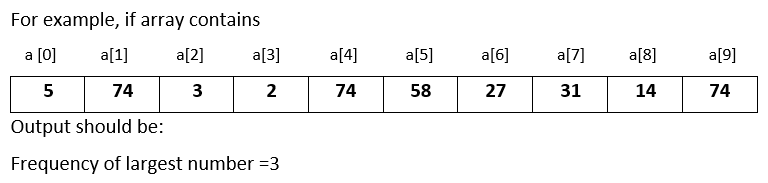# Input 10 numbers in integer array and find the frequency of the largest number in Python

###### Arrays - Question 5

In this question, we will see how to input 10 numbers in integer array and find the frequency of the largest number in Python programming. To know more about arrays click on the arrays lesson.

Q5) Write a program in Python to input 10 numbers in integer array and find the frequency of the largest number.#### Program

``````from array import *

ln=0
a=array('i',[])

print('Enter 10 numbers')
for i in range(10):
a.append(int(input()))

for i in range(10):
if i==0:
ln=a[i]

elif a[i]>ln:
ln=a[i]

print('Frequency of the Largest Number =',a.count(ln))``````

#### Output

```Enter 10 numbers
5
74
3
2
74
58
27
31
15
74
Frequency of the Largest Number = 3  ```